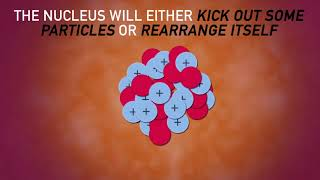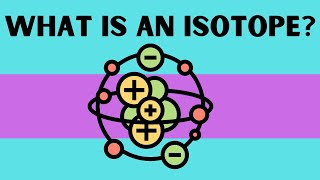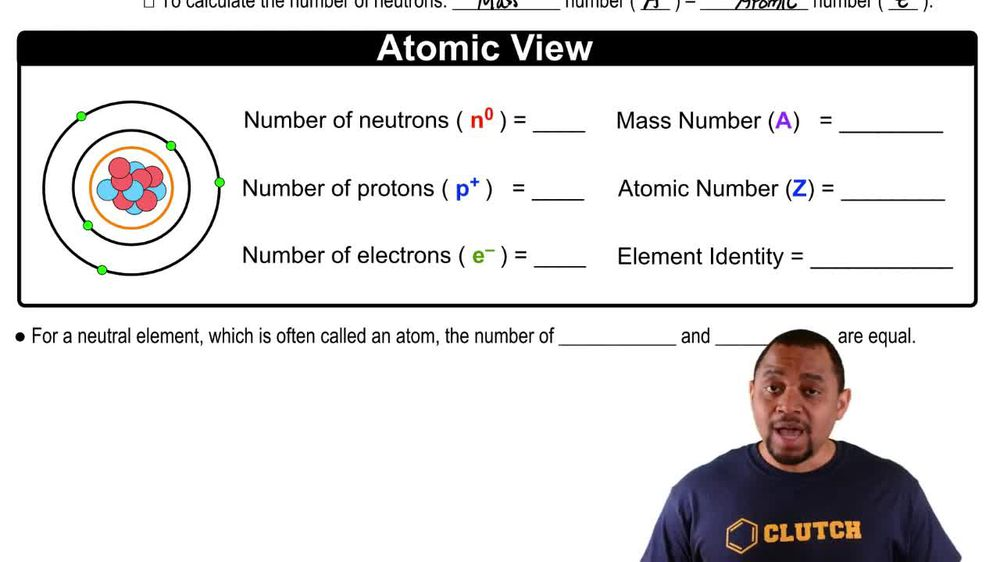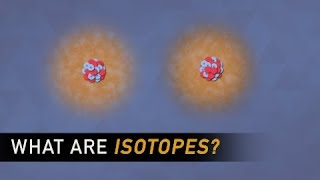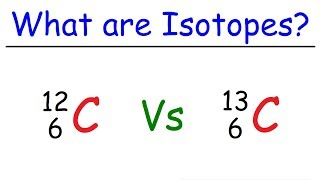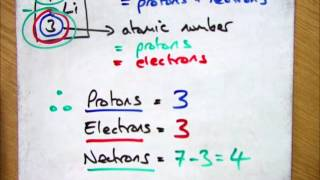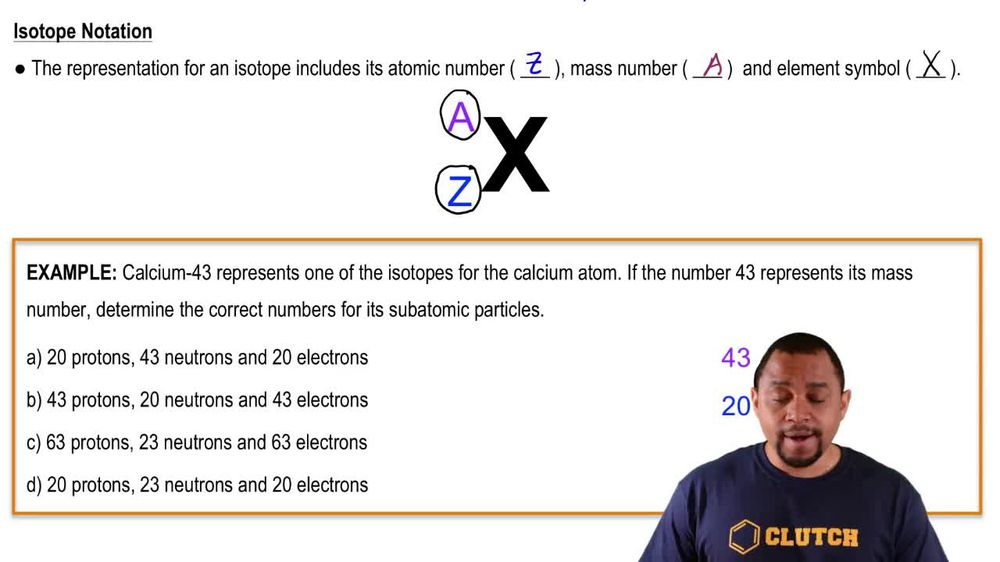Start typing, then use the up and down arrows to select an option from the list.
1. 2. Atoms & Elements2. Isotopes
Problem

# Determine the number of protons and the number of neutrons in each isotope. a. 40 19K b. 226 88Ra c. 99 43Tc d. 33 15P

Relevant Solution2m
Play a video:
hey everyone in this example, we need to figure out how many neutrons and protons are in our phosphorus isotope phosphorous, 35. We want to recall that in an isotope symbol we have X. For our element A. In the left hand superscript for our mass number. And then Z. And the left hand subscript for our atomic number. And then we want to recall that in neutral atoms, only our atomic number is going to equal our number of protons. Now we want to find our mass number from taking our number of protons and adding that to our number of neutrons. So from the symbol that were given for our phosphorous isotope, we know that A is equal to for a mass number And we know that Z is equal to 15 for our atomic number. And we also can note that we don't have a charge here on our isotope. So we can say that this is also equal to our number of protons because we have a neutral atom. and so our number of protons is equal to 15 as well as our atomic number. So we can actually box that in as our number of protons because we do have a neutral phosphorous isotope. Our next calculation is our number of neutrons. So we're going to find that by taking our mass number of 35 given in our isotope symbol here And subtracting that from our number of protons, which we found above to be 15 due to the fact that our phosphorous isotope is neutral. So when we take 35 -15, we have a difference of 20. And so we can say that we have therefore 20 neutrons, So our number of neutrons is equal to 20. So this would be our second and final answer for the number of neutrons and the number of protons in our phosphorus isotope. And this will complete this example. So if you have any questions, please leave them down below. Otherwise, I will see everyone in the next practice video.